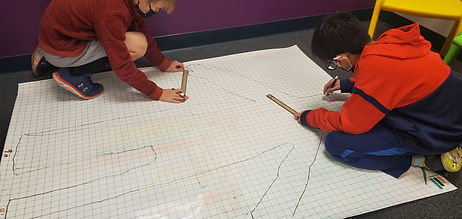Mr. Jake

Target 1​

Lesson Type:

New

Measurment

:

Techniques and Formulas

Solve problems involving perimeter.

1:

Understand that perimeter is the distance around a figure.

2:

Find the perimeter of a polygon when given all the side lengths.

3:

Solve real-world perimeter problems.

4:

Understand that the missing side can be found using the perimeter and the known sides.

5:

Find the perimeter of composite shapes.

7th

Vocabulary:

Perimeter

Activities:Home Exploration

Guiding Questions:Absent Students:

Target 2

:

1:

Convert fractions to decimals and decimals to fractions.

2:

Understand that the digit to the left of the decimal is the whole number, the digits to the right are the numerator, and the denominator is the place-value of the furthest right number.

6th

Vocabulary:

Decimal, Fraction, Convert

Activities:Home Exploration

Guiding Questions:Target 3

:

Vocabulary:

Activities:Home Exploration